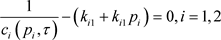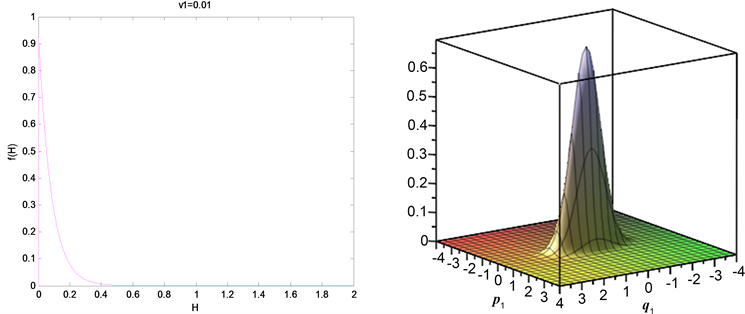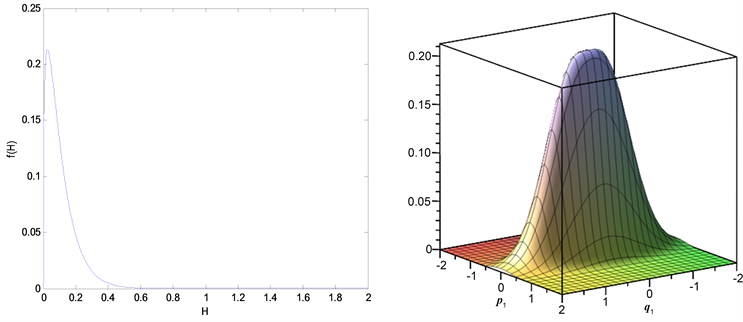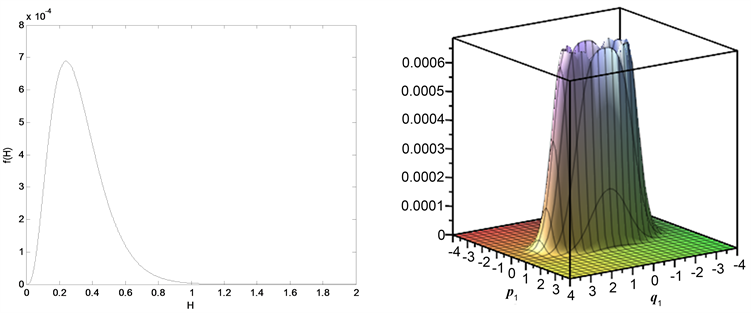# 一类转子系统的非线性随机稳定性及随机Hopf分岔Nonlinear Stochastic Stability and Stochastic Hopf Bifurcation of a Class of Rotor Systems

DOI: 10.12677/AAM.2020.94061, PDF, HTML, XML, 下载: 96  浏览: 154

Abstract: This paper mainly analyzes the nonlinear random stability and random Hopf bifurcation of a four-dimensional high-speed rotor system under a random parameter excitation. The study of rotor system dynamics has made great progress in theory and practice. The system is affected by internal factors and external random wind and replaced by Gaussian color noise. By using the principle of random average, the quasi-hamiltonian system is convergent to a one-dimensional random ITO diffusion process, and then the maximum lyapunov exponential method is used to judge the local stability of the system, and the conditions for the local stability of the system are obtained. Then the Hopf bifurcation is simulated by the solution of FPK equation, namely the stationary probability density.

1. 引言

2. 模型的建立

$\left(\begin{array}{cc}1& 0\\ 0& 1\end{array}\right)\left(\begin{array}{l}\stackrel{¨}{x}\\ \stackrel{¨}{y}\end{array}\right)+\chi \left(\begin{array}{cc}{|\stackrel{˙}{x}|}^{a}+{|x|}^{a}& 0\\ 0& {|\stackrel{˙}{y}|}^{b}+{|y|}^{b}\end{array}\right)\left(\begin{array}{l}\stackrel{˙}{x}\\ \stackrel{˙}{y}\end{array}\right)+\left(\begin{array}{cc}{k}_{1}/m& 0\\ 0& {k}_{2}/m\end{array}\right)\left(\begin{array}{l}x\\ y\end{array}\right)=\left(00\right)$

$a=b=2$，所以方程可以表示为：

$\left\{\begin{array}{l}\stackrel{¨}{x}+\chi \left({\stackrel{˙}{x}}^{2}+{x}^{2}\right)\stackrel{˙}{x}+\left({k}_{1}/m\right)x={\eta }_{1}\left(t\right)\\ \stackrel{¨}{y}+\chi \left({\stackrel{˙}{y}}^{2}+{y}^{2}\right)\stackrel{˙}{y}+\left({k}_{1}/m\right)y={\eta }_{2}\left(t\right)\end{array}$

$〈{\eta }_{i}\left(t\right)〉=0$$〈{\eta }_{i}\left(t\right){\eta }_{i}\left(s\right)〉=\frac{D}{\tau }\mathrm{exp}\left(-\frac{|t-s|}{\tau }\right)$$i=1,2$

2.1. 下面将色噪声模型进行等效白化 

$\left\{\begin{array}{l}\stackrel{¨}{x}+\chi \left({\stackrel{˙}{x}}^{2}+{x}^{2}\right)\stackrel{˙}{x}+\left(\frac{{k}_{1}}{m}\right)x={\eta }_{1}\left(t\right)\\ \stackrel{¨}{y}+\chi \left({\stackrel{˙}{y}}^{2}+{y}^{2}\right)\stackrel{˙}{y}+\left(\frac{{k}_{2}}{m}\right)y={\eta }_{2}\left(t\right)\\ {\stackrel{˙}{\eta }}_{i}\left(t\right)=-\frac{1}{\tau }{\eta }_{i}\left(t\right)+\frac{1}{\tau }{\xi }_{i}\left(t\right)\end{array}$

$x={q}_{1},\stackrel{˙}{x}={p}_{1},y={q}_{2},\stackrel{˙}{y}={p}_{2},{\omega }_{1}={k}_{1}/m,{\omega }_{2}={k}_{2}/m,\alpha =\chi$，可以得到

$\left\{\begin{array}{l}{\stackrel{˙}{q}}_{1}={p}_{1}\\ {\stackrel{˙}{p}}_{1}=-{\omega }_{1}{q}_{1}-\alpha \left({p}_{1}^{2}+{q}_{1}^{2}\right){p}_{1}+{\eta }_{1}\left(t\right)\\ {\stackrel{˙}{q}}_{2}={p}_{2}\\ {\stackrel{˙}{p}}_{2}=-{\omega }_{2}{q}_{2}-\alpha \left({p}_{2}^{2}+{q}_{2}^{2}\right){p}_{2}+{\eta }_{2}\left(t\right)\\ {\stackrel{˙}{\eta }}_{i}\left(t\right)=-\left(1/\tau \right){\eta }_{i}\left(t\right)+\left(1/\tau \right){\xi }_{i}\left(t\right)\end{array}$

${f}_{1}=-{\omega }_{1}{q}_{1}-\alpha \left({p}_{1}^{2}+{q}_{1}^{2}\right){p}_{1}$${g}_{1}=1$${f}_{2}=-{\omega }_{2}{q}_{2}-\alpha \left({p}_{2}^{2}+{q}_{2}^{2}\right){p}_{2}$${g}_{2}=1$

${\stackrel{˙}{p}}_{i}={f}_{i}+{g}_{i}\eta \left(t\right)$$i=1,2$

${\stackrel{¨}{p}}_{i}+c\left({p}_{i},\tau \right)\frac{{\stackrel{˙}{p}}_{i}}{\tau }-\frac{{f}_{i}}{\tau }=\frac{{g}_{i}\xi \left(t\right)}{\tau }$

${c}_{i}\left({p}_{i},\tau \right)=1+\tau \frac{{G}_{i}}{{g}_{i}}$ , ${G}_{i}={f}_{i}{{g}^{\prime }}_{i}-{{f}^{\prime }}_{i}{g}_{i}$ , $i=1,2$

$\left\{\begin{array}{l}{\stackrel{˙}{q}}_{1}={p}_{1}\\ {\stackrel{˙}{p}}_{1}=\frac{-{\omega }_{1}{\tau }^{-1}}{{c}_{1}\left({p}_{1},\tau \right)}{q}_{1}+\frac{-{\tau }^{-1}\alpha \left({p}_{1}^{2}+{q}_{1}^{2}\right)}{{c}_{1}\left({p}_{1},\tau \right)}{p}_{1}+\frac{{\tau }^{-1}}{{c}_{1}\left({p}_{1},\tau \right)}{\xi }_{1}\left(t\right)\\ {\stackrel{˙}{q}}_{2}={p}_{2}\\ {\stackrel{˙}{p}}_{12}=\frac{-{\omega }_{2}{\tau }^{-1}}{{c}_{2}\left({p}_{2},\tau \right)}{q}_{1}+\frac{-{\tau }^{-1}\alpha \left({p}_{2}^{2}+{q}_{2}^{2}\right)}{{c}_{2}\left({p}_{2},\tau \right)}{p}_{2}+\frac{{\tau }^{-1}}{{c}_{2}\left({p}_{2},\tau \right)}{\xi }_{2}\left(t\right)\end{array}$

$\left\{\begin{array}{l}{\stackrel{˙}{q}}_{1}={p}_{1}\\ {\stackrel{˙}{p}}_{1}=-{\tau }^{-1}\left({k}_{11}+{k}_{11}{p}_{1}\right)\left[{\omega }_{1}{q}_{1}+\alpha \left({p}_{1}^{2}+{q}_{1}^{2}\right){p}_{1}-{\xi }_{1}\left(t\right)\right]\\ {\stackrel{˙}{q}}_{2}={p}_{2}\\ {\stackrel{˙}{p}}_{2}=-{\tau }^{-1}\left({k}_{21}+{k}_{21}{p}_{2}\right)\left[{\omega }_{2}{q}_{2}+\alpha \left({p}_{2}^{2}+{q}_{2}^{2}\right){p}_{2}-{\xi }_{2}\left(t\right)\right]\end{array}$

${E}_{i}=-{\tau }^{-1}\left[\frac{1}{{c}_{i}\left({p}_{i},\tau \right)}-\left({k}_{i1}+{k}_{i1}{p}_{i}\right)\right]\left({\omega }_{i}{q}_{i}+\alpha \left({p}_{i}^{2}+{q}_{i}^{2}\right){p}_{i}-2{\xi }_{i}\left(t\right)\right),i=1,2$

$-{\tau }^{-1}\left[\frac{1}{{c}_{i}\left({p}_{i},\tau \right)}-\left({k}_{i1}+{k}_{i1}{p}_{i}\right)\right]\left({\omega }_{i}{q}_{i}+\alpha \left({p}_{i}^{2}+{q}_{i}^{2}\right){p}_{i}\right)=0,i=1,2$$\alpha {k}_{i2}{p}^{4}+\alpha {k}_{i1}{p}^{3}+\left({\tau }^{-1}{k}_{i2}+\omega {k}_{i2}+\alpha +\alpha {q}^{2}{k}_{i1}{k}_{i2}\right)p+{\tau }^{-1}{k}_{i1}+\omega {k}_{i1}+\alpha {q}^{2}{k}_{i1}-1=0$

$\left({\tau }^{-1}{k}_{i2}+\omega {k}_{i2}+\alpha \right)p+{\tau }^{-1}{k}_{i1}+\omega {k}_{i1}-1=0$

$\left\{\begin{array}{l}{\tau }^{-1}{k}_{i2}+\omega {k}_{i2}+\alpha =0\\ {\tau }^{-1}{k}_{i1}+\omega {k}_{i1}-1=0\end{array}$

$\left\{\begin{array}{l}{k}_{i1}=\frac{1}{{\tau }^{-1}+\omega }\\ {k}_{i2}=-\frac{\alpha }{{\tau }^{-1}+\omega }\end{array}$

$\left\{\begin{array}{l}{\stackrel{˙}{q}}_{1}={p}_{1}\\ {\stackrel{˙}{p}}_{1}=-{a}_{11}{q}_{1}+{b}_{11}\left({p}_{1}^{2}+{q}_{1}^{2}\right){p}_{1}+{c}_{11}{\xi }_{1}\left(t\right)\\ {\stackrel{˙}{q}}_{2}={p}_{2}\\ {\stackrel{˙}{p}}_{2}=-{a}_{22}{q}_{2}+{b}_{22}\left({p}_{2}^{2}+{q}_{2}^{2}\right){p}_{2}+{c}_{22}{\xi }_{2}\left(t\right)\end{array}$

$H=\frac{1}{2}\left({p}_{1}{}^{2}+{p}_{2}{}^{2}\right)+\frac{1}{2}\left({a}_{11}{q}_{1}^{2}+{a}_{22}{q}_{2}^{2}\right)$

$\text{d}\left(H\right)=\stackrel{¯}{m}\left(H\right)\text{d}t+\stackrel{¯}{\sigma }\left(H\right)\text{d}B\left(t\right)$

${q}_{1}=\frac{R}{\sqrt{{a}_{11}}}\mathrm{cos}\theta ,{q}_{\text{2}}=\frac{R}{\sqrt{{a}_{22}}}\mathrm{sin}\theta$

$\begin{array}{c}\stackrel{¯}{m}\left(H\right)=\frac{\text{2}\pi }{T\left(H\right)}{\int }_{\Omega }\left[\left({b}_{11}+{b}_{22}\right)A\left(H,\theta \right)-\left(\frac{{b}_{11}}{{a}_{11}}{\mathrm{cos}}^{\text{2}}\theta \text{+}\frac{{b}_{\text{22}}}{{a}_{22}}{\mathrm{sin}}^{\text{2}}\theta \right)B\left(H,\theta \right)\\ \text{\hspace{0.17em}}\text{\hspace{0.17em}}+\frac{{R}^{4}}{2}\left(\frac{{c}_{11}^{2}\pi {D}_{1}}{{a}_{11}}{\mathrm{cos}}^{2}\theta +\frac{{c}_{22}^{2}\pi {D}_{2}}{{a}_{22}}{\mathrm{sin}}^{2}\theta \right)\right]\text{d}\theta \end{array}$

${\stackrel{¯}{\sigma }}^{2}\left(H\right)=\frac{4\pi }{T\left(H\right)}{\int }_{0}^{\pi }\left(\frac{{c}_{11}^{2}\pi {D}_{1}}{{a}_{11}}{\mathrm{cos}}^{2}\theta +\frac{{c}_{22}^{2}\pi {D}_{2}}{{a}_{22}}{\mathrm{sin}}^{2}\theta \right)B\left(H,\theta \right)\text{d}\theta$

$T\left(H\right)=\left(\frac{2\pi }{\sqrt{{a}_{11}{a}_{22}}}\right){\int }_{0}^{\pi }{R}^{2}\text{d}\theta$

$\Omega =\left\{\left({Q}_{1},\cdots ,{Q}_{n},{P}_{2},\cdots ,{P}_{n}\right)|H\left({Q}_{1},\cdots ,{Q}_{n},0,{P}_{2},\cdots ,P\right)\le H\right\}$

$A\left(H,\theta \right)=H{R}^{2}-\frac{{R}^{4}}{4}\left(1+\frac{{a}_{11}+{a}_{22}}{2\sqrt{{a}_{11}{a}_{22}}}\mathrm{sin}2\theta \right)-\frac{{b}_{11}+{b}_{22}}{24}{R}^{6}{\left(\frac{\mathrm{cos}\theta }{\sqrt{{a}_{11}}}-\frac{\mathrm{sin}\theta }{\sqrt{{a}_{22}}}\right)}^{4}$

$B\left(H,\theta \right)=\frac{H{R}^{2}}{2}-\frac{{R}^{6}}{6}\left(1+\frac{{a}_{11}+{a}_{22}}{2\sqrt{{a}_{11}{a}_{22}}}\mathrm{sin}2\theta \right)-\frac{{b}_{11}+{b}_{22}}{32}{R}^{8}{\left(\frac{\mathrm{cos}\theta }{\sqrt{{a}_{11}}}-\frac{\mathrm{sin}\theta }{\sqrt{{a}_{22}}}\right)}^{4}$

${R}^{2}$ 是下列方程之解：

$H-\frac{{R}^{2}}{2}\left(1+\frac{{a}_{11}+{a}_{22}}{2\sqrt{{a}_{11}{a}_{22}}}\mathrm{sin}2\theta \right)-\frac{{b}_{11}+{b}_{22}}{8}{R}^{4}{\left(\frac{\mathrm{cos}\theta }{\sqrt{{a}_{11}}}-\frac{\mathrm{sin}\theta }{\sqrt{{a}_{22}}}\right)}^{4}=0$

2.2. 接下来主要讨论随机稳定性 

$\text{d}H={\mu }_{1}H\text{d}t+{\mu }_{2}^{1/2}H\text{d}B\left(t\right)$

${\mu }_{1}=-\frac{1}{2}\left({b}_{11}+{b}_{22}\right)+\frac{1}{2}\left(\frac{{c}_{11}^{2}{D}_{1}}{{a}_{11}}+\frac{{c}_{22}^{2}{D}_{2}}{{a}_{22}}\right)\zeta$

${\mu }_{2}=\frac{1}{3}\left(\frac{{c}_{11}^{2}{D}_{1}}{{a}_{11}}+\frac{{c}_{22}^{2}{D}_{2}}{{a}_{22}}\right)\zeta$

$\zeta ={\int }_{0}^{\pi }{\left(1+\frac{{a}_{11}+{a}_{22}}{2\sqrt{{a}_{11}{a}_{22}}}\mathrm{sin}\theta \right)}^{-2}\text{d}\theta /{\int }_{0}^{\pi }{\left(1+\frac{{a}_{11}+{a}_{22}}{2\sqrt{{a}_{11}{a}_{22}}}\mathrm{sin}\theta \right)}^{-1}\text{d}\theta$

$\stackrel{¯}{m}\left(H\right)={\mu }_{1}H+ο\left(H\right)$${\stackrel{¯}{\sigma }}^{2}\left(H\right)={\mu }_{2}{H}^{2}+ο\left(H2\right)$

$\begin{array}{l}\lambda =\underset{t\to \infty }{\mathrm{lim}}\frac{1}{t}\mathrm{ln}{H}^{\frac{1}{2}}\left(t\right)\\ \text{\hspace{0.17em}}\text{\hspace{0.17em}}\text{\hspace{0.17em}}=\frac{1}{2}\left[{m}^{\prime }\left(0\right)-{\left({\sigma }^{2}\left(0\right)\right)}^{2}\right]\\ \text{\hspace{0.17em}}\text{\hspace{0.17em}}\text{\hspace{0.17em}}=-\frac{1}{4}\left({b}_{11}+{b}_{22}\right)+\frac{1}{6}\left(\frac{{c}_{11}^{2}{D}_{1}}{{a}_{11}}+\frac{{c}_{22}^{2}{D}_{2}}{{a}_{22}}\right)\zeta \end{array}$

$\frac{2}{3}\left(\frac{{c}_{11}^{2}{D}_{1}}{{a}_{11}}+\frac{{c}_{22}^{2}{D}_{2}}{{a}_{22}}\right)\zeta <\left({b}_{11}+{b}_{22}\right)$

3. 随机分岔分析

$\frac{\partial p}{\partial t}=-\frac{\partial }{\partial H}\left[a\left(H\right)p\right]+\frac{1}{2}\frac{{\partial }^{2}}{\partial {H}^{2}}\left[b\left(H\right)p\right]$

$a\left(H\right)=\stackrel{¯}{m}\left(H\right),b\left(H\right)={\stackrel{¯}{\sigma }}^{2}\left(H\right)$

${\alpha }_{H}=1$${\beta }_{H}=2$

${c}_{H}=\underset{x\to {x}_{H}^{+}}{\mathrm{lim}}\frac{2a\left(x\right){\left(x-{x}_{H}\right)}^{{\alpha }_{H}-{\beta }_{H}}}{b\left(x\right)}=-\frac{3}{2\zeta }\left({b}_{11}+{b}_{22}\right)+\frac{3}{2}\left(\frac{{c}_{11}^{2}{D}_{1}}{{a}_{11}}+\frac{{c}_{22}^{2}{D}_{2}}{{a}_{22}}\right)$

$f\left(H\right)=C{H}^{{v}_{1}}\mathrm{exp}\left[{u}_{1}H\right]$

${v}_{1}={c}_{H}-{\alpha }_{H}=-2+\left(-\frac{3}{2\zeta }\left({b}_{11}+{b}_{22}\right)+\frac{3}{2}\left(\frac{{c}_{11}^{2}{D}_{1}}{{a}_{11}}+\frac{{c}_{22}^{2}{D}_{2}}{{a}_{22}}\right)\right)$

${u}_{1}=\frac{3}{2}\left(\frac{{c}_{11}^{2}{D}_{1}}{{a}_{11}}+\frac{{c}_{22}^{2}{D}_{2}}{{a}_{22}}\right)$

$\begin{array}{l}f\left({q}_{1},{q}_{2},{p}_{1},{p}_{2}\right)=C{\left(\frac{1}{2}\left({p}_{1}^{2}+{p}_{2}^{2}\right)+\frac{1}{2}\left({a}_{11}{q}_{1}^{2}+{a}_{22}{q}_{2}^{2}\right)\right)}^{{v}_{1}}\\ \text{\hspace{0.17em}}\text{\hspace{0.17em}}\text{\hspace{0.17em}}\text{\hspace{0.17em}}\text{\hspace{0.17em}}\text{\hspace{0.17em}}\text{\hspace{0.17em}}\text{\hspace{0.17em}}\text{\hspace{0.17em}}\text{\hspace{0.17em}}\text{\hspace{0.17em}}\text{\hspace{0.17em}}\text{\hspace{0.17em}}\text{\hspace{0.17em}}\text{\hspace{0.17em}}\text{\hspace{0.17em}}\text{\hspace{0.17em}}\text{\hspace{0.17em}}\text{\hspace{0.17em}}\text{\hspace{0.17em}}\text{\hspace{0.17em}}\text{\hspace{0.17em}}\text{\hspace{0.17em}}\text{\hspace{0.17em}}\text{\hspace{0.17em}}×\mathrm{exp}\left[{u}_{1}\left(\frac{1}{2}\left({p}_{1}^{2}+{p}_{2}^{2}\right)+\frac{1}{2}\left({a}_{11}{q}_{1}^{2}+{a}_{22}{q}_{2}^{2}\right)\right)\right]\end{array}$

4. 数值模拟Figure 1. When ${b}_{11}+{b}_{22}=-9.67$ , ${v}_{1}=0.01$ system stationary probability density function and joint probability density functionFigure 2. When ${b}_{11}+{b}_{22}=-9.89$ , ${v}_{1}=0.335$ system stationary probability density function and joint probability density functionFigure 3. When ${b}_{11}+{b}_{22}=-11.67$ , ${v}_{1}=3$ system stationary probability density function and joint probability density function

5. 小结

  Xiang, L., Hu, A.J., Hou, L.L., Xiong, Y.P. and Xing, J.T. (2016) Nonlinear Coupled Dynamics of an Asymmetric Double-Disc Rotor-Bearing System under Rub-Impact and Oil-Fi1m Forces. Applied Mathematical Modelling, 40, 4505-4523. https://doi.org/10.1016/j.apm.2015.11.028  吕延军, 虞烈, 刘恒. 非线性轴承–转子系统的稳定性和分岔[J]. 机械工程学报, 2004, 40(10): 62-67.  杨乐昌, 张建国, 孙京, 韩建超. 考虑参数随机分布特性的碰摩转子动力学与可靠性[J]. 航空动力学报, 2014, 29(8): 1961-1967.  Harish Chandra, N. and Sckhar, A.S. (2016) Nonlinear Damping Identification in Rotors Using Wavelet Transform. Mechanism and Machine Theory, 100, 170-183. https://doi.org/10.1016/j.mechmachtheory.2016.02.007  Tasker, F. and Chopra, I. (1992) Nonlinear Damping Estimation from Rotor Stability Data Using Time and Frequency Domain Techniques. AIAA Journal, 30, 1383-1391. https://doi.org/10.2514/3.11074  严惠云. 非线性经济模型的动力学行为研究[D]: [博士学位论文]. 西安: 西北工业大学, 2017.  朱位秋. 随机振动[M]. 北京: 科学出版社, 1998.  朱位秋. 非线性随机动力学与控制—Hamilton理论体系框架[M]. 北京: 科学出版社, 2003.